# 14-240/Tutorial-October7

## Boris

#### Subtle Errors in Proofs

Check out these proofs:

##### Proof 1

Let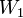$W_1$,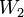$W_2$ be subspaces of a vector space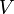$V$.

We show that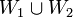$W_1 \cup W_2$ is a subspace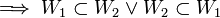$\implies W_1 \subset W_2 \or W_2 \subset W_1$.

Assume that$W_1 \cup W_2$ is a subspace.
Let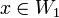$x \in W_1$,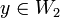$y \in W_2$.
Then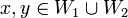$x, y \in W_1 \cup W_2$.
Then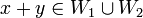$x + y \in W_1 \cup W_2$.
Then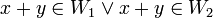$x + y \in W_1 \or x + y \in W_2$.
Case 1: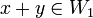$x + y \in W_1$:
Since$x \in W_1$ and$W_1$ has additive inverses, then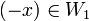$(-x) \in W_1$.
Then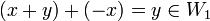$(x+y)+(-x)=y \in W_1$.
Case 2:$x + y \in W_2$:
Since$y \in W_2$ and$W_2$ has additive inverses, then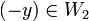$(-y) \in W_2$.
Then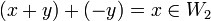$(x+y)+(-y)=x \in W_2$.
Then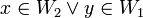$x \in W_2 \or y \in W_1$.
Then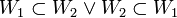$W_1 \subset W_2 \or W_2 \subset W_1$. Q.E.D.
##### Proof 2

Let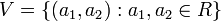$V=\{(a_1, a_2):a_1, a_2 \in R\}$. Then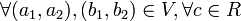$\forall (a_1, a_2), (b_1, b_2) \in V, \forall c \in R$, define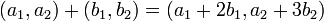$(a_1, a_2) + (b_1, b_2) = (a_1 + 2b_1, a_2 + 3b_2)$ and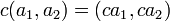$c(a_1, a_2)=(ca_1, ca_2)$.

We show that$V$ is not a vector space over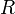$R$.

We show that$V$ is not commutative.
Let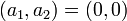$(a_1, a_2) = (0, 0)$.
Then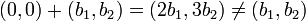$(0, 0) + (b_1, b_2) = (2b_1, 3b_2) \neq (b_1, b_2)$.
Then$V$ is not commutative.
Then$V$ is not a vector space. Q.E.D.

Can you spot the subtle error in each?

In Proof 1, the equivalence of (2) the last line and (1) the "let" statement to the second last line is not obvious:

(1) Let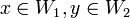$x \in W_1, y \in W_2$. [Many lines] Then$x \in W_2 \or y \in W_1$.

(2) Then$W_1 \subset W_2 \or W_2 \subset W_1$.

Rewrite sentences (1) and (2) into a form that is easier to compare:

(1)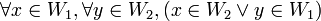$\forall x \in W_1, \forall y \in W_2, (x \in W_2 \or y \in W_1)$.

(2)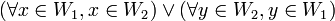$( \forall x \in W_1, x \in W_2) \or ( \forall y \in W_2, y \in W_1)$.

For Proof 1 to be correct, we must show that sentences (1) and (2) are equivalent. Alternatively, alter the structure of Proof 1 into a proof by contradiction.

In Proof 2, the only thing that is shown is that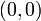$(0, 0)$ is not the additive identity. For Proof 2 to be correct, either plug in a vector that is not$(0, 0)$ or show that$(0, 0)$ is the additive identity by some other means, which introduces a contradiction.